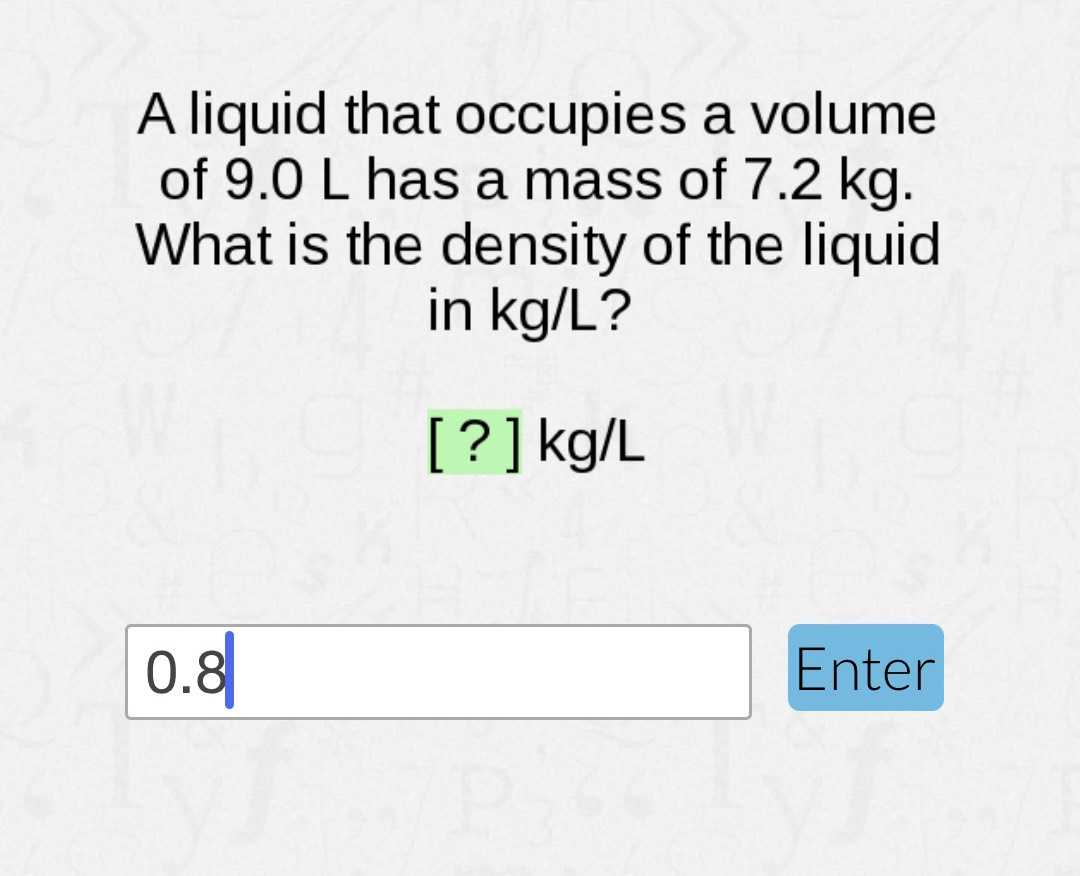### ¿Todavía tienes preguntas de matemáticas?

Pregunte a nuestros tutores expertos
Algebra
PreguntaA liquid that occupies a volume of $$9.0$$ L has a mass of $$7.2 kg$$ . What is the density of the liquid in $$kg / L$$ ?

$$0.8\ kg/L$$